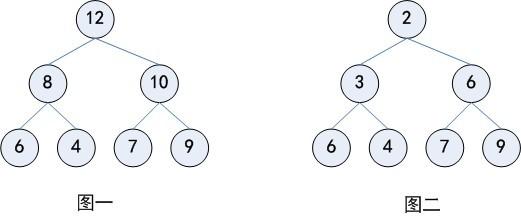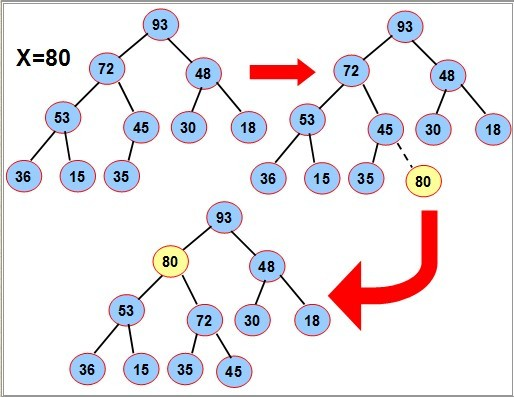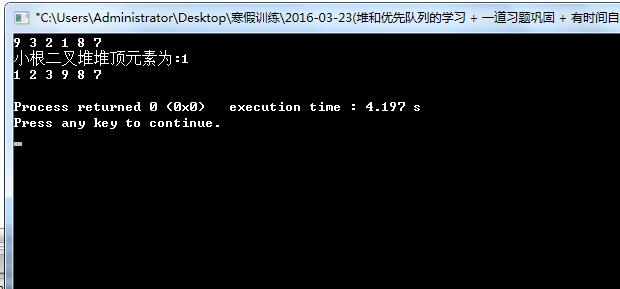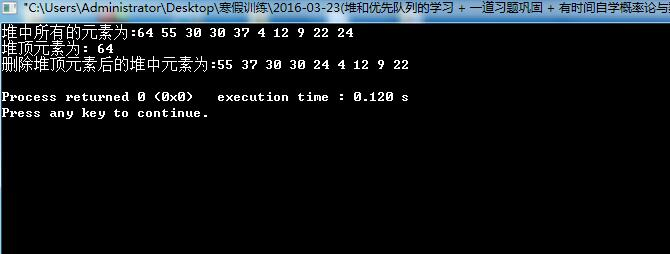# 堆的概念及基本操作实现

1.堆的基本概念：2.堆的一些基本性质：

（1）. 堆的插入和删除操作，运行时间为 O(logn)，n 为树上结点的个数

2^x - 1 = n；

（2）.堆可以看成是一棵完全二叉树，除最后一层其余每层结点都是满的。

3.堆的插入和删除操作实现：

（1）.首先我们看堆的插入操作：（1）.左孩子节点的编号是其自身节点编号 * 2 + 1；

（1）.右孩子节点的编号是其自身节点编号 * 2 + 2；（编号是指其用数组中存储时的下标）

//往堆中插入元素,数组下标从0开始

void push(int value){   //value表示插入堆的值
Heap[size] = value;    //一开始元素接在堆的最后面
int current = size;      //表示当前遍历到的节点
int father = (current-1) / 2;    //表示当前遍历到的堆中元素父亲节点的下标
while(current > 0 && Heap[current] < Heap[father]){
swap(Heap[current],Heap[father]);       //表示此时节点"上溢",当前节点与其父节点对换.
current = father;
father = (current-1) / 2;     //继续向上遍历
}
++size;
}
（2）.其次是删除操作：

//获取堆顶元素
int top(){
return Heap;
}

/**删除栈顶元素
*删除栈顶元素时,不可直接将size的值减一后就结束,这样的话会破坏整个
*二叉堆的结构,因此我们在删除堆顶元素之后还要调整堆的结构,使其成为新的堆
*并且有新的堆顶元素
*/
void pop(){
Heap = Heap[--size];     /*首先将最后一个元素替换堆顶元素,这样的话堆顶元素则被删除
*然后再是size减一表示堆中元素数目减一
*/
int current = 0;            //当前遍历到的节点的下标
int lchild = current*2 + 1,rchild = current*2 + 2;  //当前遍历到的节点的左孩子和右孩子节点的下标
while(lchild < size && min(Heap[lchild],Heap[rchild]) < Heap[current]){
if(Heap[lchild] < Heap[rchild]){
swap(Heap[lchild],Heap[current]);
current = lchild;       //"下溢"的过程
}
else{
swap(Heap[rchild],Heap[current]);
current = rchild;
}
lchild = current*2 + 1;
rchild = current*2 + 2;
}

}

【算法】堆，最大堆（大顶堆）及最小堆（小顶堆）的实现

        //二叉堆(数组存储)的插入,删除堆顶元素(以小根堆为例,即堆顶元素最小)
#include<cstdio>
#include<algorithm>
using namespace std;
const int Max_size = (int)1e3;  //表示堆中能够容纳元素个数的最大值
int size = 0,Heap[Max_size];         //记录当前堆中元素下标最大值
//往堆中插入元素,数组下标从0开始

void push(int value){   //value表示插入堆的值
Heap[size] = value;    //一开始元素接在堆的最后面
int current = size;      //表示当前遍历到的节点
int father = (current-1) / 2;    //表示当前遍历到的堆中元素父亲节点的下标
while(current > 0 && Heap[current] < Heap[father]){
swap(Heap[current],Heap[father]);       //表示此时节点"上溢",当前节点与其父节点对换.
current = father;
father = (current-1) / 2;     //继续向上遍历
}
++size;
}

/**删除栈顶元素
*删除栈顶元素时,不可直接将size的值减一后就结束,这样的话会破坏整个
*二叉堆的结构,因此我们在删除堆顶元素之后还要调整堆的结构,使其成为新的堆
*并且有新的堆顶元素
*/
void pop(){
Heap = Heap[--size];     /*首先将最后一个元素替换堆顶元素,这样的话堆顶元素则被删除
*然后再是size减一表示堆中元素数目减一
*/
int current = 0;            //当前遍历到的节点的下标
int lchild = current*2 + 1,rchild = current*2 + 2;  //当前遍历到的节点的左孩子和右孩子节点的下标
while(lchild < size && min(Heap[lchild],Heap[rchild]) < Heap[current]){
if(Heap[lchild] < Heap[rchild]){
swap(Heap[lchild],Heap[current]);
current = lchild;       //"下溢"的过程
}
else{
swap(Heap[rchild],Heap[current]);
current = rchild;
}
lchild = current*2 + 1;
rchild = current*2 + 2;
}

}

//获取堆顶元素
int top(){
return Heap;
}

//输出堆中元素
void output(){
for(int i = 0;i < size;++i){
printf("%d ",Heap[i]);
}
printf("\n");
}
int main(){
int a;
for(int i = 0;i < 6;++i){
scanf("%d",&a[i]);
push(a[i]);
}
printf("小根二叉堆堆顶元素为:%d\n",top());
output();
return 0;
}C++实现的大根堆的插入和删除操作：

#include<iostream>
using namespace std;
class Heap {
private:
int *data, size;
public:
Heap(int length_input) {
data = new int[length_input];
size = 0;
}
~Heap() {
delete[] data;
}
void push(int value) {
data[size] = value;
int current = size;
int father = (current - 1) / 2;
while (data[current] > data[father]) {
swap(data[current], data[father]);
current = father;
father = (current - 1) / 2;
}
size++;
}
void output() {
for (int i = 0; i < size; i++) {
cout << data[i] << " ";
}
cout << endl;
}
int top(){
return data;
}
void update(int pos,int n){
int lchild = 2 * pos + 1,rchild = 2 * pos + 2;
int max_value = pos;
if(lchild < n && data[lchild] > data[max_value]){
max_value = lchild;
}
if(rchild < n && data[rchild] > data[max_value]){
max_value = rchild;
}
if(max_value != pos){
swap(data[pos],data[max_value]);
update(max_value,n);
}
}
void pop(){
swap(data,data[size-1]);
--size;
update(0,size);
}
};
int main() {
int arr = { 12, 9, 30, 24, 30, 4, 55, 64, 22, 37 };
Heap heap(100);
for (int i = 0; i < 10; i++) {
heap.push(arr[i]);
}
cout << "堆中所有的元素为:";
heap.output();
cout <<"堆顶元素为: " << heap.top() << endl;
heap.pop();         //删除堆顶元素
cout << "删除堆顶元素后的堆中元素为:";
heap.output();
return 0;
}05-13133307-128679
08-263174
01-30134
05-152323
02-173828
04-125278
05-26227
11-181147
05-021669
12-101万+
11-13166
04-11378
07-111万+
05-12663
07-031656
01-21116
05-15406
05-17308